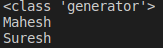# Decorators and Generators in Python

## Decorators and Generators in Python with Examples

In this article, I am going to discuss Decorators and Generators in Python with Examples. Please read our previous article where we discussed Recursive and Lambda Functions in Python with examples. As part of this article, we are going to discuss the following pointers which are related to Decorators and Generators in Python.

1. Decorators in Python
2. @ symbol in python
3. Generators in Python
4. next function in Python
##### Decorators in Python with Examples:

We have already discussed nested functions and function as first class object concepts already. Clear understanding of these concepts is important in understanding decorators. A decorator is a special function which adds some extra functionality to an existing function. The meaning of the statement can be understood clearly by the end of this topic. For now let’s understand a decorator as:

1. A decorator is a function that accepts a function as a parameter and returns a function.
2. Decorators are useful to perform some additional processing required by a function.
##### Steps to create decorator:

Step1: Decorator takes a function as an argument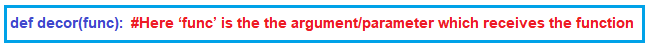Step2: Decorator body should have an inner functionStep3: Decorator should return a functionStep4: The extra functionality which you want to add to a function can be added in the body of the inner_function.

Let’s create a function which takes two arguments and prints the sum of them.

```def add(a,b):
res = a + b
return res

###### Output: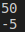Now, I wish to add some extra functionality of adding the two numbers only if they are positive. If any number is negative, then I wish to take it as 0 during adding. For adding this extra functionality let create a decorator.

```def decor(func):  #Here ‘func’ is the the argument/parameter which receives the function
def inner_function(x,y):
if x<0:
x = 0
if y<0:
y = 0
return func(x,y)
return inner_function  #Decor returns the func passed to it.```

We have created our decorator and now let’s use it with our add function from demo40.py

With the above statement, we are passing the add function as parameter to the decorator function, which is returning inner_function. Now, the inner_function object or address will be overridden in the ‘add’ because we are capturing the returned function in it. After this, whenever we call add, the execution goes to inner_function in the decorator.

In inner_function, we are doing the extra logic for checking whether the arguments are positive or not. If not positive we are assigning them with zero. And we are passing the processed values to the original add function which was sent to the decorator. Our final code will be

###### Example: Decorator Function in Python (Demo41.py)
```def decor(func):
def inner_function(x,y):
if x<0:
x = 0
if y<0:
y = 0
return func(x,y)
return inner_function

res = a + b
return res

###### Output: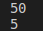##### @ symbol in python:

In the above example, in order to use the decorator, we have used the ‘add = decor(add)’ line. Rather than using this we can just use the ‘@decor’ symbol on top of the function for which we want to add this extra functionality. The decorator once created can also be used for other functions as well.

###### Example: Subtract Function using decorator function in Python (Demo40.py)
```def decor(func):
def inner_function(x,y):
if x<0:
x = 0
if y<0:
y = 0
return func(x,y)
return inner_function

@decor
def sub(a,b):
res = a - b
return res

print(sub(30,20))
print(sub(10,-5))```
###### Output: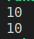##### Generators in Python with Examples:

Generators are just like functions which give us a sequence of values one as an iterable (which can be iterated upon using loops). Generators contain yield statements just as functions contain return statements.

###### Example: Generators in Python (Demo41.py)
```def m():
yield 'Mahesh'
yield 'Suresh'

g = m()
print(g)
print(type(g))
for y in g:
print(y)```
###### Output: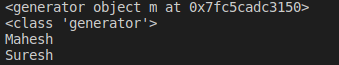###### Example: Generators in Python (Demo42.py)
```def m(x, y):
while x<=y:
yield x
x+=1

g = m(5, 10)
for y in g:
print(y)```
###### Output: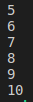##### next function in Python:

If we want to retrieve elements from a generator, we can use the next function on the iterator returned by the generator. This is the other way of getting the elements from the generator. (The first way is looping in through it as in the examples above).

###### Example: Generators with next function in Python (Demo43.py)
```def m():
yield 'Mahesh'
yield 'Suresh'
g = m()

print(type(g))
print(next(g))
print(next(g))```
###### Output: